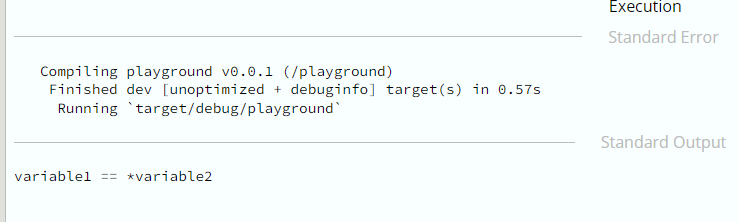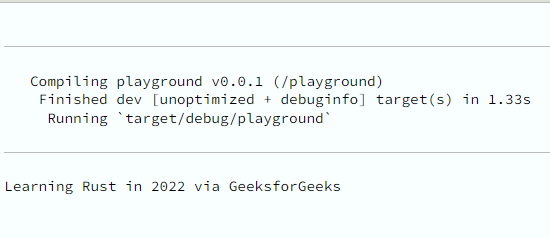Open in App
Not now

# Rust – Deref Trait

• Last Updated : 15 Sep, 2022

Deref<T> trait in Rust is used for customizing dereferencing operator (*) behavior. Implementing the Deref <T> treats the smart pointer as a reference. Therefore, any code that references the deref trait would also refer to smart pointers.

### Regular References in Deref Trait:

Regular reference in Rust is a pointer that points to a value stored in a different memory location.  In this example, we see cite the example of two variables – variable1 and variable2. Then, we create a reference as well as dereference to demonstrate regular references use in the Deref trait.

Example 1:

## Rust

 `// Rust code for Deref Trait ` ` `  `fn` `main()   ` `{   ` `  ``let` `variable1 = 100;   ` `  ``let` `variable2 = &variable1;   ` `  ``if` `variable1==*variable2   ` `  ``{   ` `    ``println!(``"variable1 == *variable2"``);   ` `  ``}   ` `  ``else`   `  ``{   ` `    ``println!(``"variable1 != *variable2"``);   ` `  ``}   ` `}`

Output:### Deref Trait Implementation:

Deref trait is a library in Rust that implements the deref method. Deref is used for dereferencing operations as we did in this example (*variable 2). We create a structure GFG which is a generic type (T) and then implemented the Deref trait.

Example 2:

## Rust

 `// Rust code for Deref Trait ` ` `  `struct` `GFG   ` ` ``{   ` `  ``gfg_variable : T,   ` ` ``}   ` `  ``use` `:: std::ops::Deref;   ` ` `  `  ``impl`` Deref ``for` `GFG   ` ` ``{   ` `  ``type` `Target = T;   ` `  ``fn` `deref(&``self``) ->&T   ` `   ``{   ` `     ``&``self``.gfg_variable   ` `   ``}   ` ` ``}   ` ` `  `fn` `main()   ` `{   ` `  ``let` `variable = GFG{gfg_variable : 2022};   ` `  ``print!(``"Learning Rust in {} via GeeksforGeeks"``, ` `    ``*(variable.deref()));   ` `}`

Output:Explanation:

Here:

• the GFG struct type is being implemented by Deref Trait
• gfg_variable is the variable returned by the deref() method after the deref trait is implemented.
• Generally, associated types are used to declare the generic type parameter, and type target =T is declared as an associated type for the Deref trait.

My Personal Notes arrow_drop_up
Related Articles